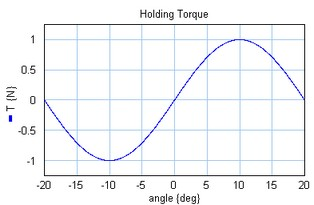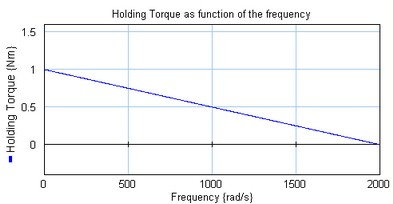﻿ 20-sim webhelp > Library > Iconic Diagrams > Mechanical > Rotation > Actuators > Steppermotor

# Steppermotor

## Library

Iconic Diagrams\Mechanical\Rotation\Actuators

## Use

Domains: Continuous. Size: 1-D. Kind: Iconic Diagrams (Rotation).

## Description

A stepper motor is a device used to convert electrical pulses into discrete mechanical rotational movements. The minimum movement, invoked by one pulse, is called the step angle. The electrical pulses are mostly generated by a pulse generator that converts a setpoint change in a corresponding amount of pulses. This model describes a combination of a pulse generator and stepper motor.

At standstill the torque required to deflect the motor a full step is called the holding torque. The holding torque normally is much higher than required to drive the load, and thus acts as a strong brake to hold the load. The holding torque as function of the output angle is shown in the graph below. Around zero angle the curves shows the behavior of a spring. By changing the input by one step, the force will increase to its maximum and move the the load.Motor torque a s function of the angle (holding toque = 1 [N], step angle = [10°] ).

If the subsequent steps are generated fast enough the holding torque will start to decrease. The maximum holding torque as function of the rotation speed is shown in the figure below. This force curve is commonly describe as the pull out curve.Pull out curve: maximum generated torque as a function of the rotational speed.

 Ports Description input p_out Desired output angle. Rotation port. Causality fixed current out p_in preferred angular velocity out p_out Inputs input Stepper motor input Parameters step_angle tau Th J B fmax Minimum change of the output axis [deg]. Time constant coil [s]. Holding torque [Nm]. Inertia rotor [Nm^2] Relative damping at the stepper motor resonance []. Maximum frequency motor (frequency at which the torque gets zero) [rad/s].